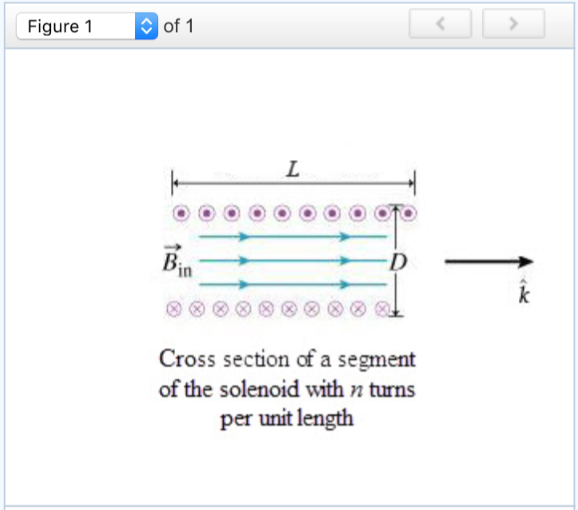# Problem: Magnetic Field inside a Very Long Solenoid Learning Goal: To apply Ampère's law to find the magnetic field inside an infinite solenoid. In this problem we will apply Ampère's law to calculate the magnetic field inside a very long solenoid (only a relatively short segment of the solenoid is shown in the pictures). The segment of the solenoid shown in (Figure 1) has length L, diameter D, and n turns per unit length with each carrying current I. It is usual to assume that the component of the current along the z axis is negligible. (This may be assured by winding two layers of closely spaced wires that spiral in opposite directions.) From symmetry considerations it is possible to show that far from the ends of the solenoid, the magnetic field is axial.Part DWhat is Iencl, the current passing through the chosen loop?Express your answer in terms of L (the length of the Ampèrean loop along the axis of the solenoid) and other variables given in the introduction.Part EFind Bin, the z component of the magnetic field inside the solenoid where Ampère's law applies.Express your answer in terms of L, D, n, I, and physical constants such as ?0.

###### FREE Expert Solution

Part D

The magnetic field due to a solenoid in a rectangular path,

${\mathbf{\oint }}{\mathbf{B}}{\mathbf{·}}{\mathbf{ds}}{\mathbf{=}}{\mathbf{\oint }}{\mathbf{B}}{\mathbf{·}}{\mathbf{ds}}{\mathbf{+}}{\mathbf{\oint }}{\mathbf{B}}{\mathbf{·}}{\mathbf{ds}}{\mathbf{+}}{\mathbf{\oint }}{\mathbf{B}}{\mathbf{·}}{\mathbf{ds}}{\mathbf{+}}{\mathbf{\oint }}{\mathbf{B}}{\mathbf{·}}{\mathbf{ds}}$

When the magnetic field and area integral are perpendicular,

Thus for the rectangular path,

$\begin{array}{rcl}{\mathbf{\oint }}{\mathbf{B}}{\mathbf{·}}{\mathbf{ds}}& \mathbf{=}& {\mathbf{\oint }}{\mathbf{B}}{\mathbf{·}}{\mathbf{ds}}{\mathbf{+}}{\mathbf{\oint }}{\mathbf{B}}{\mathbf{·}}{\mathbf{ds}}{\mathbf{+}}{\mathbf{\oint }}{\mathbf{B}}{\mathbf{·}}{\mathbf{ds}}{\mathbf{+}}{\mathbf{\oint }}{\mathbf{B}}{\mathbf{·}}{\mathbf{ds}}\\ & \mathbf{=}& \mathbf{0}\mathbf{+}\mathbf{0}\mathbf{+}\mathbf{B}\mathbf{L}\mathbf{+}\mathbf{0}\end{array}$

${\mathbf{\oint }}{\mathbf{B}}{\mathbf{·}}{\mathbf{ds}}{\mathbf{=}}{\mathbit{B}}{\mathbit{L}}$

From amperes circuit law,

$\overline{)\begin{array}{rcl}{\mathbf{BL}}& {\mathbf{=}}& {\mathbf{\mu }}_{\mathbf{0}}\mathbf{NI}\end{array}}$

$\begin{array}{rcl}\mathbf{B}\mathbf{L}& \mathbf{=}& {{\mathbf{\mu }}}_{{\mathbf{0}}}{\mathbf{NI}}\\ \mathbf{B}& \mathbf{=}& {{\mathbf{\mu }}}_{{\mathbf{0}}}{\mathbf{·}}\frac{\mathbf{N}}{\mathbf{L}}{\mathbf{·}}{\mathbf{I}}\\ & \mathbf{=}& {{\mathbf{\mu }}}_{{\mathbf{0}}}{\mathbf{nI}}\end{array}$

92% (14 ratings)###### Problem DetailsMagnetic Field inside a Very Long Solenoid Learning Goal: To apply Ampère's law to find the magnetic field inside an infinite solenoid. In this problem we will apply Ampère's law to calculate the magnetic field inside a very long solenoid (only a relatively short segment of the solenoid is shown in the pictures). The segment of the solenoid shown in (Figure 1) has length L, diameter D, and n turns per unit length with each carrying current I. It is usual to assume that the component of the current along the z axis is negligible. (This may be assured by winding two layers of closely spaced wires that spiral in opposite directions.) From symmetry considerations it is possible to show that far from the ends of the solenoid, the magnetic field is axial.

Part D

What is Iencl, the current passing through the chosen loop?

Express your answer in terms of L (the length of the Ampèrean loop along the axis of the solenoid) and other variables given in the introduction.

Part E

Find Bin, the z component of the magnetic field inside the solenoid where Ampère's law applies.

Express your answer in terms of LDnI, and physical constants such as ?0.